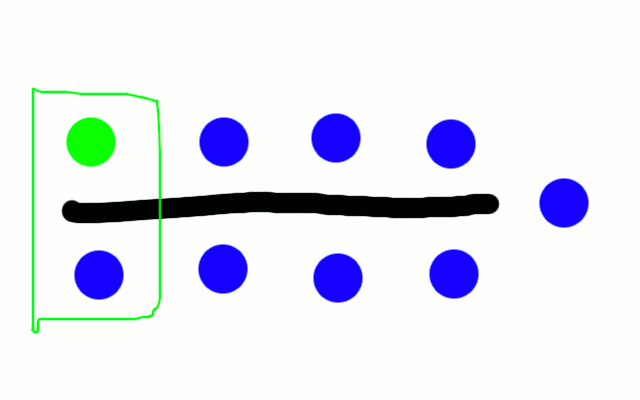# Pair up!

Published on 2017-04-21 math

Recently I stumbled upon a question that tickled my mathematical senses. It seemed simple enough but not too simple:

I was in a workshop situation where we wanted to repeatedly split into pairs until everyone had talked to everyone. How could this be done? Measures of quality of the algorithm would be:

• Should be finished after a minimal number of rounds.
• No person should be singled out.
• It should be simple to explain what everyone has to do.

## First approach: Lower bound

In a group of `n` people, there are `n * (n - 1) / 2` pairs in total. At one time, there can be at most `floor(n / 2)` pairs. So if `n` is even the minimal number of rounds is `n - 1`, if it is odd it is `n`. This makes sense because each person has to speak to `n - 1` people.

## Second approach: Divide and conquer

The problem can be solved with a simple recursive algorithm:

Split into two groups of size `floor(n / 2)` and `ceil(n / 2)`. Have everyone from the one group talk to everyone from the other. This will take `ceil(n / 2)` rounds. Then repeat for each individual group until only one person is left per group.

In each iteration, there can be one additional round necessary due to the rounding. In the worst case, this takes `n - 1 + log2(n - 1)` rounds.

This algorithm is simple, but not optimal. Let's look at the example of `n = 6`:

``````1-4 2-5 3-6
1-5 2-6 3-4
1-6 2-4 3-5
1-2 4-5
1-3 4-6
2-3 5-6``````

In the later rounds we have people waiting who could pair up. We should skip the first round instead.

## Optimal solution: Distances

Let's first concentrate on the case where `n` is odd.

You have a row of tables with one pair each. On the end of a round, everyone moves to their left (clockwise). Because both you and the people on the other side of the table move, you skip over one person.

On one end of the row of tables, you switch to the other side directly, so that you talk to people with an odd distance to your right from then on. On the other end, you have to skip one round, so that you talk to people with an even distance.

If `n` is even, the whole thing will execute as if `n` was actually `n - 1` and you talk to person `n` instead of skipping a round on the end of the row.Here is an implementation of this algorithm in python:

``````def pairs(n):
is_even = n % 2 == 0

if is_even:
n = n - 1

for _round in range(n):
r = []
for i in range(int((n + 1) / 2)):
r.append([])

for person in range(n):
table = (_round + person) % n
if table > n / 2:
table = n - table
r[table].append(person)

if is_even:
r.append(n + 1)

print(r)``````

## Conclusion

The second algorithm actually is optimal in terms of time. It is also relatively easy to understand. The only issue is that, in the even case, person `n` has a special role.

I would like to see how well this works in practice.